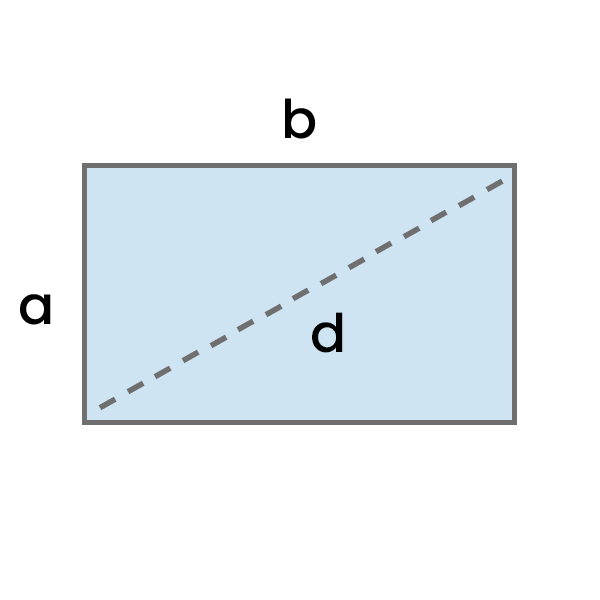# Diagonal of a Rectangle Calculator

Calculate Diagonal of a Rectangle based on the entered length and width in selected units, and see the step-by-step calculation process.Result:

## How to calculate Diagonal of a Rectangle?

To calculate the diagonal of a rectangle, add the square of its height to the square of its width, and then take the square root of the result.

Diagonal of a Rectangle = √(a² + b²)
• a - Height
• b - Width
Example: Calculate the diagonal of a rectangle with height of 4 cm and width of 7 cm.

Diagonal = √(a² + b²)

Diagonal = √(16 cm² + 49 cm²)

Diagonal = √(65 cm²)

Diagonal = 8.06 cm